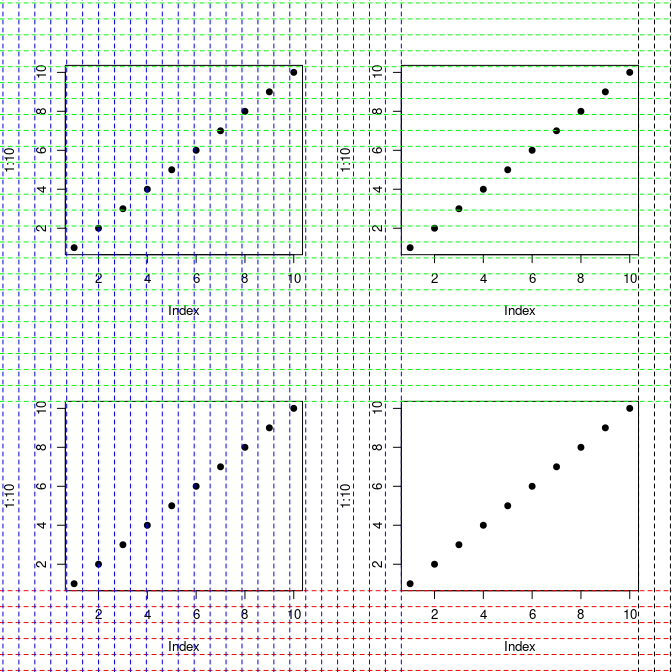# Using the precisePlacement Package

#### 2021-06-14

This package provides a selection of tools that make it easier to place elements onto a (base R) plot exactly where you want them. It provides translations between inches, pixels, margin lines, data units, and proportions of the plotting space so that elements can easily be added relative to each other or to the figure boundaries.

The “How big is your graph?” cheat sheet written by Steve Simon, found at https://www.rstudio.com/wp-content/uploads/2016/10/how-big-is-your-graph.pdf, was very helpful. If you find yourself referring to that often then perhaps this package may be of interest.

## Conventions

All four-unit values are returned in the order of (bottom, left, top, right) like par('mar') and not like par('usr') which is (left, right, bottom, top).

All two-unit values are returned in the order of (x-axis, y-axis) like par('pin').

## Preliminaries: The Device Region and Margin Lines

Plots created with base R contain four different “regions”. The first is the innermost rectangle that the data points are displayed in. I refer to this as the “data region”. By default this is extended by 4% in both the x and y axes before the axis labels are added. This is the “plot region”. This behaviour can be disabled using the parameters xaxs = 'i' and yaxis = 'i', either passed to par() or to plot(). If so the plot region will be the same size as the data region. Next is the “figure region”. This includes the plot region plus the margin lines given by par(mar). By default this is c(5, 4, 4, 2) + 0.1; if set to zero for all four sides then the figure region will be the same size as the plot region. Finally there is the device region. This contains the figure region(s) plus any outer margin lines. There are none by default, meaning the device region is the same size as the figure region for single-panel plots, but outer margins can be specified using par(oma).

The plot below illustrates these regions. The functions used to highlight the regions and lines can be useful for diagnostic purposes.

library(precisePlacement)

par(xpd = NA, oma = 1:4)

plot(1:10, pch = 19)

highlightDeviceRegion()
highlightFigureRegion()
highlightPlotRegion()
highlightDataRegion()

showMarginLines()
showOuterMarginLines()

legend('topleft', c('Data Region', 'Plot Region', 'Figure Region', 'Device Region'),
text.col = c('darkgreen', 'red', 'orange', 'skyblue'), bty = 'n', text.font = 2,
xjust = 0.5, yjust = 0.5)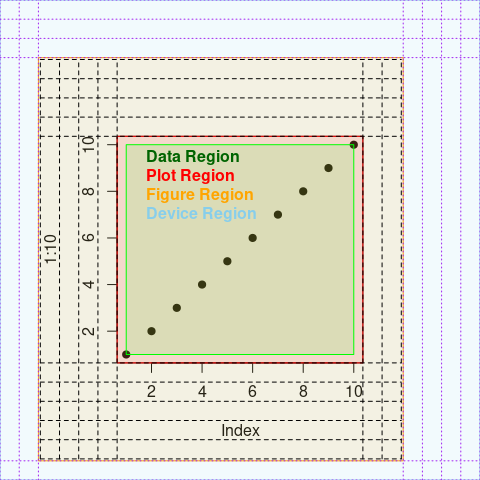These illustrations make it easier to judge what value is needed for the line parameter in the likes of axis, mtext, rug, and many more. There is also the function lineLocations to help with this, which returns the coordinates of each margin line in the same units as the data axes.

## Plot Coordinates

The regions of a plot can be measured in units of inches, pixels, margin lines, or data (meaning whatever is being plotted).

The getBoundaries function will return the four corners of either the data, plot, figure, or device region, in units of either data or margin lines. (This is similar to par('usr') which returns the boundaries of the plot region in units of data.)

The getRange function will return the distance between the left and right boundaries and the bottom and top boundaries. Again this can be for the data, plot, figure, or device region but in addition to data and margin lines it can also give units of inches or pixels.

## Unit Conversions

For relative conversions between inches, pixels, margin lines, and data units there are a number of functions of the form “getXperY” as shown in the example below. These return numeric vectors of length two giving the value for the x and y axes, respectively.

plot(1:10)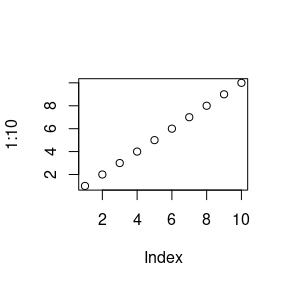getLinesPerInch()
#>  5 5
getInchesPerLine()
#>  0.2 0.2
getDataPerLine()
#>  1.104545 1.675862
getLinesPerDatum()
#>  0.9053498 0.5967078
getDataPerInch()
#>  5.522727 8.379310
getInchesPerDatum()
#>  0.1810700 0.1193416
getPixelsPerInch()
#>  96 96
getInchesPerPixel()
#>  0.01041667 0.01041667
getPixelsPerLine()
#>  19.2 19.2
getLinesPerPixel()
#>  0.05208333 0.05208333
getPixelsPerDatum()
#>  17.38272 11.45679
getDataPerPixel()
#>  0.05752841 0.08728448

For absolute conversions we can use the convertUnits function which will transform the units of a specific point on a plot. In this case the possible units are data points, margin lines, and proportions of the data/plot/figure/device region.

plot(seq(as.Date('2018-01-01'), as.Date('2019-01-01'), length.out = 10), 1:10,
pch = 19, xlab = '', ylab = '')

## Identify the center of the plot.
abline(h = convertUnits('proportion', 0.5, 'data', axis = 'y'),
col = 'red', lwd = 4)
abline(v = convertUnits('proportion', 0.5, 'data', axis = 'x'),
col = 'blue', lwd = 4)

## Change the region we are defining the proportions from.
abline(v = convertUnits('proportion', 0.75, 'data', axis = 'x', region = 'plot'),
col = 'darkgreen', lwd = 4)
abline(v = convertUnits('proportion', 0.75, 'data', axis = 'x', region = 'device'),
col = 'orange', lwd = 4)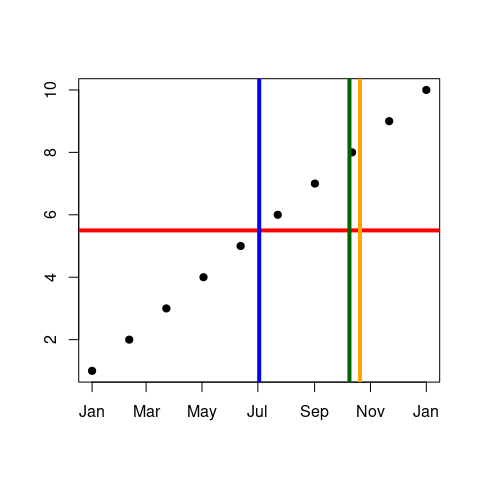This is useful if we want to place a legend somewhere specific.

par(xpd = NA, oma = c(0, 0, 0, 5))
plot(1:10, pch = 19)

legend(x = 11,
y = convertUnits('proportion', 0.5, 'data', axis = 'y', region = 'device'),
LETTERS[1:8], ncol = 1, bty = 'n', text.font = 2, text.col = 1:8,
xjust = 0.5, yjust = 0.5, cex = 3
)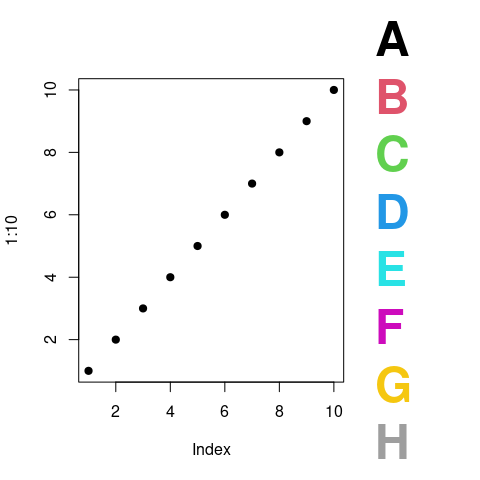We can also use the relative unit conversions to easily size elements for plotting. Below is a contrived example where we create a simple timeline chart - note how easy it is to select a value for lwd so that we don’t have to use rect.

projects <- list(A = as.Date(c('2018-01-01', '2018-06-04')),
B = as.Date(c('2018-02-01', '2018-11-01')),
C = as.Date(c('2018-11-01', '2018-12-01')),
D = as.Date(c('2018-03-01', '2018-05-01')),
E = as.Date(c('2018-01-01', '2018-03-01')),
F = as.Date(c('2018-09-01', '2018-12-01')),
G = as.Date(c('2018-05-01', '2018-07-01'))
)

x <- seq(as.Date('2018-01-01'), as.Date('2019-01-01'), length.out = length(projects))
y <- 1:length(projects)

plot(x, y, xlim = range(x), ylim = c(0.5, length(projects) + 0.5),
type = 'n', xlab = 'Date', ylab = '', axes = FALSE, xaxs = 'i', yaxs = 'i')

axis(1, pretty(x), pretty(x))
axis(2, at = y, labels = names(projects), las = 2)
box()

lineWidth <- (getRange('plot', 'data') / length(projects)) * getPixelsPerDatum()

for (ii in seq_along(projects))
lines(projects[[ii]], rep(ii, 2), lwd = lineWidth, ljoin = 2, lend = 1)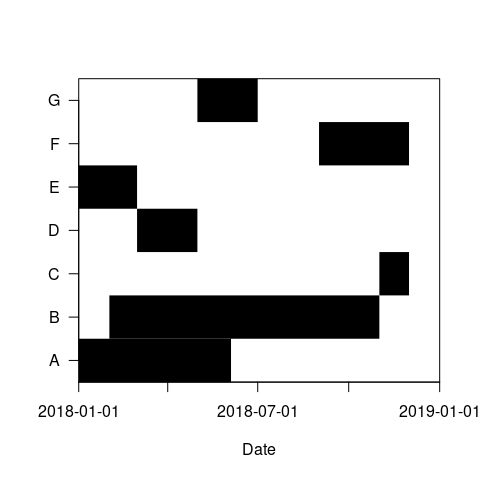## Subfigures

The omiForSubFigure function allows users to easily create a vector of four values with units of inches to pass to par('omi') and create a new plot as a sub-figure of an existing one.

plot(1:10, pch = 19)

originalPar <- par()

## Select a region in terms of proportions of the existing one.
omi <- omiForSubFigure(0.5, 0.05, 0.95, 0.5, region = 'device')
par(omi = omi, new = TRUE, xpd = NA)
plot(1:10, pch = 19, col = 'red')
highlightFigureRegion()

## Reset to the original par otherwise we will be referencing the subplot.
originalPar[c('cin', 'cra', 'csi', 'cxy', 'din', 'page')] <- NULL
par(originalPar)

## Select a new region in terms of the original plotting units.
omi <- omiForSubFigure(2, 6, 5, 10, units = 'data')
par(omi = omi, mar = c(0, 0, 0, 0))
plot(1:10, pch = 19, col = 'red', xlab = '', ylab = '')
highlightFigureRegion()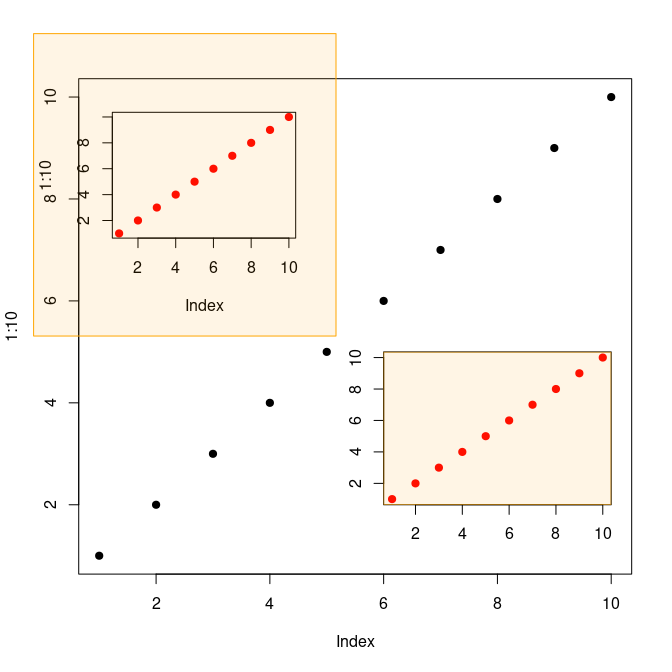Note that when specifying the proportions for omiForSubFigure the top and right sides are defined with reference to the bottom and left. This is because I found it more intuitive to specify, for example, the fraction of the plot between 0.4 and 0.7 rather than counting 0.4 from one side and 0.3 from the other.

## Multiple Plots Per Device

All of the functions mentioned above can also be used in cases of multiple plots per device. Those that pertain to only one of the plots will apply to the currently active one as per par('mfg').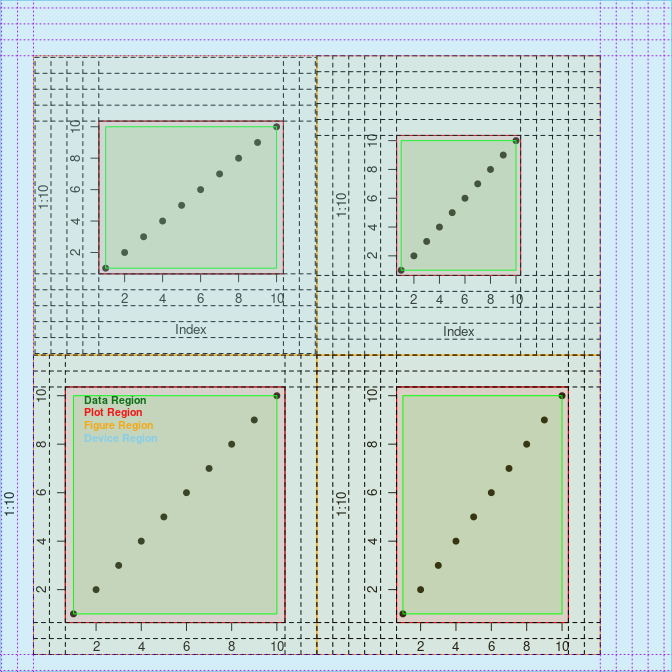#> par('mfg'):  2 2 2 2
#> Boundaries of data region: 1 1 10 10
#> Boundaries of plot region: 0.64 0.64 10.36 10.36
#> Boundaries of figure region: -0.6749051 -3.866536 11.67491 12.16261
#> Boundaries of device region: -1.398103 -21.78843 26.3258 16.2185
par(xpd = NA, mfrow = c(2, 2))

plot(1:10, pch = 19)
plot(1:10, pch = 19)
plot(1:10, pch = 19)
plot(1:10, pch = 19)

print(getBoundaries('device', units = 'data'))
#>   -3.531204 -15.806265  30.957847  11.731374
print(getBoundaries('device', units = 'lines'))
#>   5.10000 25.18434 25.18434  2.10000

x <- getBoundaries('device', units = 'lines')
## lineLocations is a shortcut to using convertUnits.
abline(h = lineLocations(side = 1, 0:x), col = 'red', lty = 2)
abline(v = lineLocations(side = 2, 0:x), col = 'blue', lty = 2)
abline(h = lineLocations(side = 3, 0:x), col = 'green', lty = 2)
abline(v = lineLocations(side = 4, 0:x), col = 'black', lty = 2)